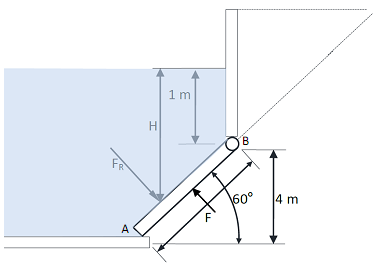## Determine the pressure point for hydrostatic force, Mechanical Engineering

Assignment Help:

The 300 kg, 8-m-wide rectangular gate shown in the figure is hinged at B and leans against the floor at A making an angle of 60° with the horizontal. The gate is to be open from its lower edge by applying force a normal force at its centre. Determine the minimum force, F required to open the gate and determine the pressure point for the hydrostatic force.#### The aligned dimension command, The Aligned Dimension Command You can us...

The Aligned Dimension Command You can use this command to generate aligned dimensions. These are dimensions along inclined lines which cannot be dimensioned with the DIMLINEAR

#### Define the primitives of b-rep scheme, Primitives of b-rep Scheme The b...

Primitives of b-rep Scheme The building blocks for b-rep. models are : Vertex It is a unique point (an ordered triplet) in space. Edge An edge is a finite, non-

#### Determine the mean radius of an open coiled spring, Determine the mean radi...

Determine the mean radius of an open coiled spring: Determine the mean radius of an open coiled spring of helix angle of 38 o , to give a vertical displacement of 20 mm and an

#### Computer integrated manufacturing, components of cim

components of cim

#### Determine the stresses acting on the plan, Using Mohr's Circle Method deter...

Using Mohr's Circle Method determine the stresses acting on the plan as shown in figure. Also write the procedure.

#### Continuous and salt bath furnaces, Continuous Furnace: Parts to be tr...

Continuous Furnace: Parts to be treated are situated on several sort of conveyor that move into the furnaces as per to programmed heating cooling cycle. The time for unloadin

#### Tool cost, what is tool cost?

what is tool cost?

#### Pendulum pump mechanism, describe about pendulum pump mechanism and dia...

describe about pendulum pump mechanism and diagram

#### Determine the relative maximum error, a) Round off the numbers 865250 amd 3...

a) Round off the numbers 865250 amd 37.46235 to four significant figures and compute Ea, Er, Ep in each case. b) IF u= 4 x 2 y 3 /z 4 and errors in x, y, z be 0.001, determine

#### Design a helical spring, A semi elliptical spring has 10 leaves in all, wit...

A semi elliptical spring has 10 leaves in all, with the two full length leaves extending 625 mm. It is 62.5 mm wide and is prepared by strips 6 mm thick. Prepare a design of helica